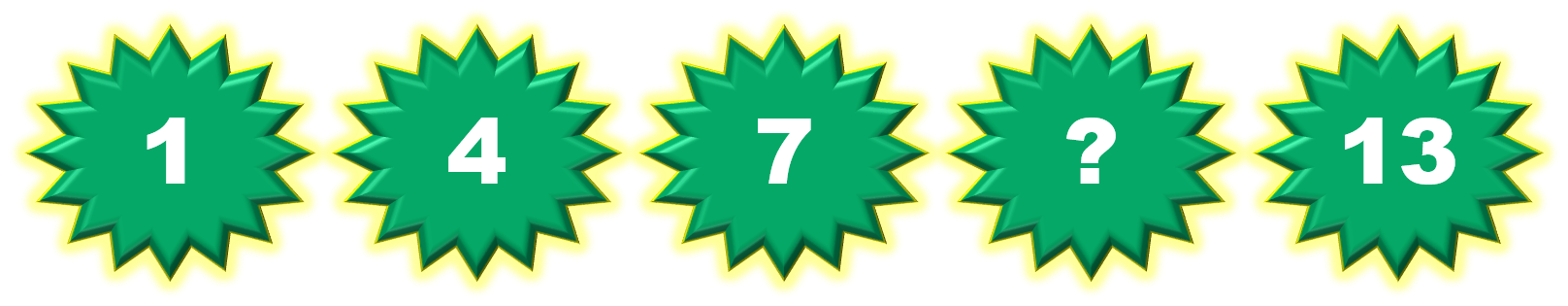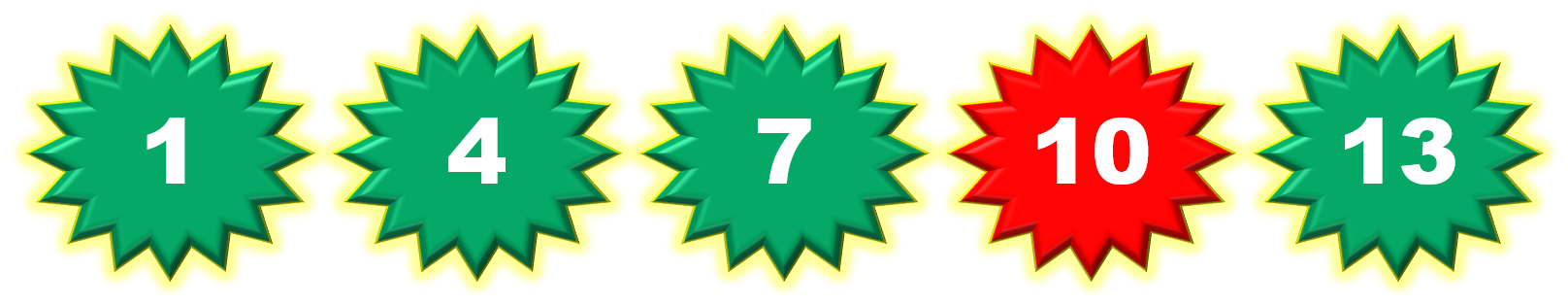1
visibility

Consider the given number pattern and identify the missing number.• A

8

• B

9

• C

10

• D

11

Solution:

Considering the given number pattern.Here, the numbers are increasing.
So, to find the missing number, we first find the rule.

Note that:
1 + 3 = 4
4 + 3 = 7
7 + 3 = 10
10 + 3 = 13

So, the rule is "add 3".Then, the missing number is 10.